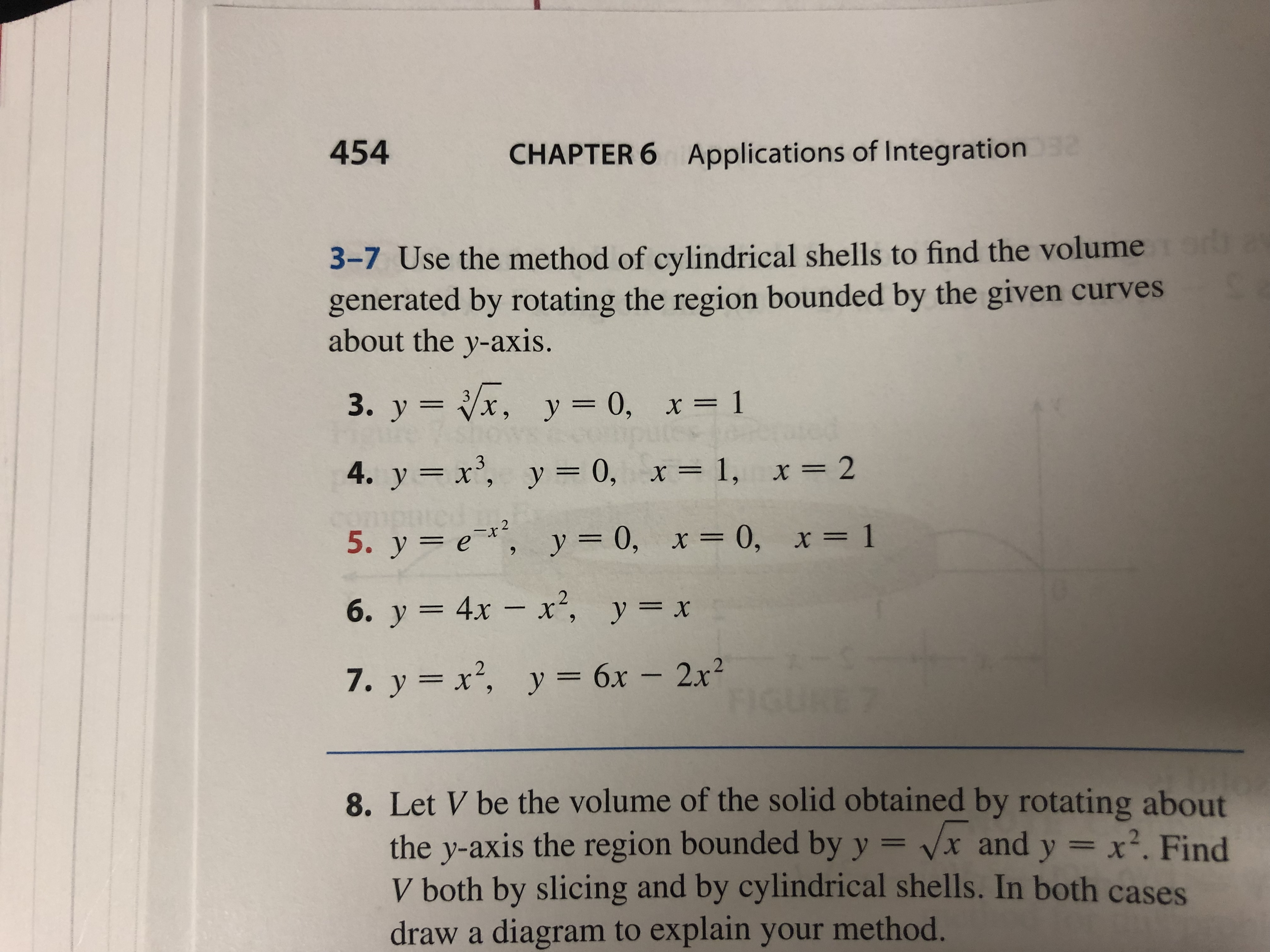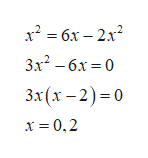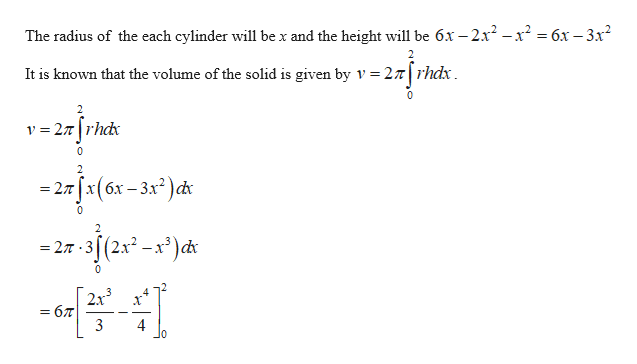# 454CHAPTER 6Applications of Integration23-7 Use the method of cylindrical shells to find the volumegenerated by rotating the region bounded by the given curvesabout the y-axis.3. y x, y = 0, x= 14. y x, y = 0, x= 1, x = 225. у %3D е*, у 3D0, х3D 0, х%3D 16. у 3 4х — х*, у%3D х7. y x2, y 6x - 2x28. Let V be the volume of the solid obtained by rotating aboutthe y-axis the region bounded by y = vx and y x2. FindV both by slicing and by cylindrical shells. In both casesdraw a diagram to explain your method.

Question
3 views

#7help_outlineImage Transcriptionclose454 CHAPTER 6 Applications of Integration 2 3-7 Use the method of cylindrical shells to find the volume generated by rotating the region bounded by the given curves about the y-axis. 3. y x, y = 0, x= 1 4. y x, y = 0, x= 1, x = 2 2 5. у %3D е*, у 3D0, х3D 0, х%3D 1 6. у 3 4х — х*, у%3D х 7. y x2, y 6x - 2x2 8. Let V be the volume of the solid obtained by rotating about the y-axis the region bounded by y = vx and y x2. Find V both by slicing and by cylindrical shells. In both cases draw a diagram to explain your method. fullscreen
check_circle

Step 1

It is given that the curves are f(x) = x2 and g(x) = 6x–2x2 bounded about the y-axis.

Find the intersection of the two curves by equating each other as follows.help_outlineImage Transcriptioncloseх? 3 бх - 2х? 3x2 — бх %3D 0 Зx(х - 2)-0 х%3D0,2 fullscreen
Step 2

Integrate the function using the cylindrical...help_outlineImage TranscriptioncloseThe radius of the each cylinder will be x and the height will be 6x - 2xr2-x2 = 6x - 3x2 It is known that the volume of the solid is given by V 27rhdx 0 2 v 27rhd = 0 - 2л (x (бх - 3х?)ф 2 (2x2 -x3)cd* = 27 2x3 = 67 3 4 fullscreen

### Want to see the full answer?

See Solution

#### Want to see this answer and more?

Solutions are written by subject experts who are available 24/7. Questions are typically answered within 1 hour.*

See Solution
*Response times may vary by subject and question.
Tagged in

### Other